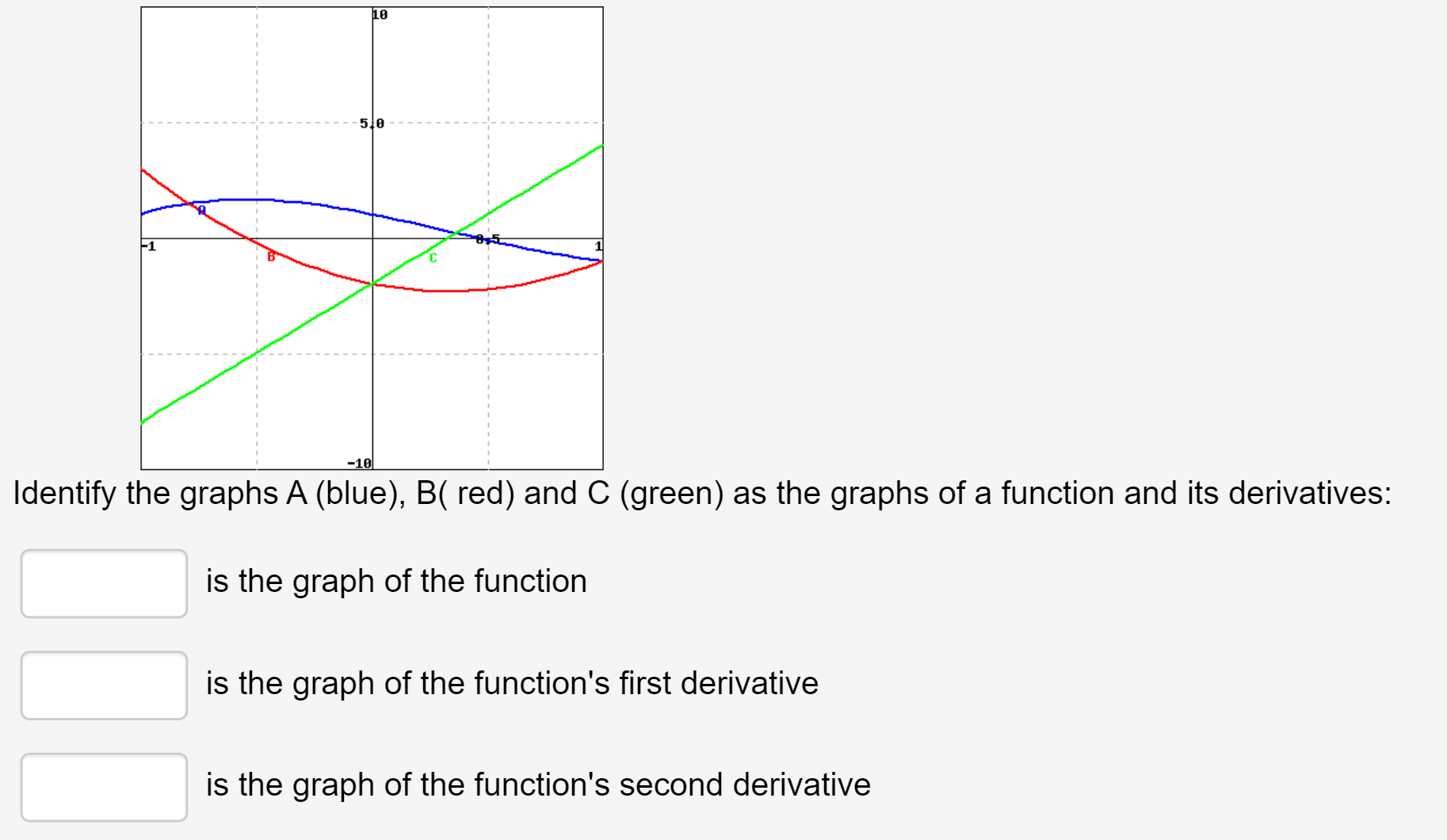# 10 5 0 -1 -10 Identify the graphs A (blue), B( red) and C (green) as the graphs of a function and its derivatives: is the graph of the function is the graph of the function's first derivative is the graph of the function's second derivative

Questionhelp_outlineImage Transcriptionclose10 5 0 -1 -10 Identify the graphs A (blue), B( red) and C (green) as the graphs of a function and its derivatives: is the graph of the function is the graph of the function's first derivative is the graph of the function's second derivative fullscreen

1 Rating

### Want to see the step-by-step answer?